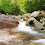## August 17, 2016

### Accurate temperature reading from Raspberry PI Sense HAT

When I first received the Sense HAT for my Raspberry PI 3 I decided to start building a nice weather station using the embedded environmental sensors and RGB LED matrix.
From a quick experiment it turns out that the temperature readings are not accurate. The problem is caused by thermal conduction from the Pi CPU to the humidity and pressure sensors on the Sense HAT.
I have experimented few algorithms and I have found the following formula to be the most reliable. It basically compensate the temperature reading (t) with the CPU temperature (tCpu).
tCorr = t - ((tCpu-t)/1.5)

Here is the full script to print each 5 seconds the environmental data including the corrected temperature.

```import os
import time
from sense_hat import SenseHat

def get_cpu_temp():
t = float(res.replace("temp=","").replace("'C\n",""))
return(t)

sense = SenseHat()

while True:
t = sense.get_temperature_from_humidity()
t_cpu = get_cpu_temp()
h = sense.get_humidity()
p = sense.get_pressure()

# calculates the real temperature compesating CPU heating
t_corr = t - ((t_cpu-t)/1.5)

print("t=%.1f  t_cpu=%.1f  t_corr=%.1f  h=%d  p=%d" % (t, t_cpu, t_corr, round(h), round(p)))

time.sleep(5)
```

Running this script I have noticed that the CPU temperature reading is not very stable making the corrected temperature a little bit unstable. To fix this issue I have decided to apply a moving average to the temperature reading.
As a further improvement I'm also the temperature both from the humidity and pressure sensors and calculating the average.

```import os
import time
from sense_hat import SenseHat

# get CPU temperature
def get_cpu_temp():
t = float(res.replace("temp=","").replace("'C\n",""))
return(t)

# use moving average to smooth readings
def get_smooth(x):
if not hasattr(get_smooth, "t"):
get_smooth.t = [x,x,x]
get_smooth.t = get_smooth.t
get_smooth.t = get_smooth.t
get_smooth.t = x
xs = (get_smooth.t+get_smooth.t+get_smooth.t)/3
return(xs)

sense = SenseHat()

while True:
t1 = sense.get_temperature_from_humidity()
t2 = sense.get_temperature_from_pressure()
t_cpu = get_cpu_temp()
h = sense.get_humidity()
p = sense.get_pressure()

# calculates the real temperature compesating CPU heating
t = (t1+t2)/2
t_corr = t - ((t_cpu-t)/1.5)
t_corr = get_smooth(t_corr)

print("t1=%.1f  t2=%.1f  t_cpu=%.1f  t_corr=%.1f  h=%d  p=%d" % (t1, t2, t_cpu, t_corr, round(h), round(p)))

time.sleep(5)
```

1.Interesting, I thought the problem was with the sensor being so close to the board. I will have to try your solution.

2.How hard would it be to add more sensors and cobble it all together to send to wunderground?

1.It would be simple but costly, the Sense HAT is mainly useful for its 8x8 LED Matrix and its humidity and pressure sensors

3.Wouldn't it be better to model the "heat transfer" conditions and then apply the right formula?

4.My Sense hat give me humidity readings of 120℅ !!! and pressure of around 16 milibar lower than reference weather stations.

5.Thank you so much for sharing this information with us. Your post is full of information. I like your idea of sharing this post.

Thermocouple Sheath

6.The formula used in your code is great for a RPI3 , could you give me some hint for changing it for the Raspberry zero W temperatures? (using the RPI3 formula the temperatures are too low).

7.This is cool, but the sense has the same inaccurate temperature even if you have it 12 inches away from the Pi3. The problem is clearly, and sadly, not from the Pi3 CPU.

8.9.Nice blogs...
Thanks for sharing...
Humidity Meter, Controller, Data logger, pH meter

10.I would like to utilize the Pi to collect water pressure data and temperature. Could I do that?

11.I have simply bought a mini black hat hack3r from Pimoroni and keep the SenseHAT 10cm apart from the Pi - problem solved...

1.this is the best solution from my opinion

12.13.I have just gone through your blog......your information is so valuable. Im Aarudhra from Chennai Thermocouple Sensor